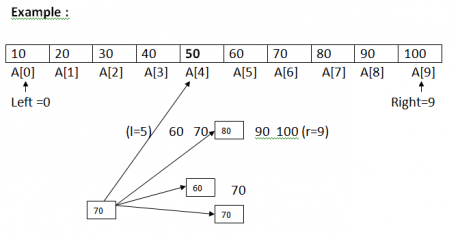# Binary search in Data Structures

21 September, 2018

Ratings

## Binary search

The binary search in data structures is yet another simple method. However in the binary search, the algorithm expect the list to be sorted you can apply any one of the sorting methods before using the binary search algorithm. In binary search the given list is devided into two equal half’s. The given key is compared with the middle element of the list now three situations may occur. The middle element matches with the key the search will end peacefully here. The middle element is greater than the key then the value which we are searhing is possibly in the first half of the list. The middle element is lower than the key. Then the value which were searching is possibly in the second half of the list   Now the list is searched either in the first half is in the second half of got by the result of conditions (b) and (c) given above. This search fails because the key does not existMid= left+right/2 Mid= 0+9/2 = 4.5   Program Binary search # include <stdio.h> # include <conio.h> void main() \{ int a,n,key,p,i; clrscr(); printf(“Enter the total number of elements \n”); scanf(“%d”,&n); printf(“Enter the element in ascending order \n”); for(i=0;i<n;i++) { scanf(“%d”,&a[i]); } printf(“Enter the search element \n”); scanf(“%d”,&key); p=binary search(a,n,key);     /* Functional */ if(p>=0) { printf(“search is successful \n”); printf(“The element found at position %d”,p+1); } else { printf(“search is unsuccessful search \n”); } else { printf (“search is unsuccessful search \n”); } } int binary search(int a,int n, int key) { int left, right, mid; left=0; right=n-1; while(left<=right) { mid=(left+right)/2; if(key==a[mid]) { right=mid-1; } else { left=mid+1; } } } return(-1); }   Time complexity: Each step at the algorithm divides the list divided into two parts and the search continues in one of them and the other is discarded the search requires at mast k steps where 2k > =N which results k=log2N thus the running time c both average and worst case of binary search is proportional